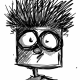# Python: How to Write a dance() Function in BlenderLast week, I wrote a script to pull objects in Blender into the shape of an H and I. But after running it with a couple different sets of objects, the text seemed a bit bland. After everything finds a place, it just sits there. I’d like it to twirl around a little. So I wrote it into a function—dance().

## Why Make It A Function?

Making the text dance around is pretty simple. But it’s repetitive. For each light, I want to set a keyframe, then move everything a tad at random, then set another keyframe, then move everything a tad again, then set another keyframe.

Putting those 10 lines of code into a function means I can make multiple things in my script dance by just typing in dance().

## But How Do You Make the Function?

It’s actually pretty simple.

def nameoffunction():
lines of code to run when function is called

Most of the work goes into figuring out what you want the function to do.

Using random.uniform() , I can get the computer to randomize numbers for the x, y, and z values. Inside the parenthesis, I put a maximum and minimum to make sure the text didn’t go flying off too far. For my purposes, I figured it shouldn’t move more than maybe .06 between keyframes. Setting it to a range of -.03 and .03 meant that the text wouldn’t float off into the positive axes uniformly. I put that value into a variable for easier adjustments in the future. That got me here.Plug it into a Halloween scene and run it on a bunch of selected objects (like lights)—and you’ll get something like this:What’s really cool about making functions is that you can put variables inside the parentheses (that bit is called a parameter), and it’ll let you customize elements of the function when you call it.

For instance, if you move devVal between the parentheses and write dance(.03), you’ll get the same result.A slightly more complicated example got me the Happy Halloween text above.

In this script, I made sets of coordinates for lines (middle, right, left, top, and bottom). Then assembled those sets into letters. Then defined a function to print the text based on the letter set and make it dance around a bit. Here’s the copy/paste version with some comment explanations:

```
import bpy
import random

#Sets all lights to 0 at frame 0
for obj in bpy.context.selected_objects:
obj.location.x=0
obj.location.y=0
obj.location.z=-1.7
obj.keyframe_insert(data_path="location",frame=0)

#Explodes lights out randomly at frame 60
for obj in bpy.context.selected_objects:
obj.location.x=random.uniform(-15,15)
obj.location.y=random.uniform(0,15)
obj.location.z=random.uniform(-2,15)
obj.keyframe_insert(data_path="location",frame=60)
obj.keyframe_insert(data_path="location",frame=110)

selObj = []
#BEFORE STARTING YOU HAVE TO SELECT ALL OBJECTS
#THIS CREATES AN LIST OF SELECTED OBJECTS
for obj in bpy.context.selected_objects:
selObj.append(obj.name)

x=0
y=-2
z=0
h=1
w=.5
midLine = [[x,y,z+(.5*h)],[x+(.85*w),y,z+(.5*h)],[x+(.75*w),y,z+(.5*h)],[x+(.50*w),y,z+(.5*h)],[x+(.25*w),y,z+(.5*h)],[x+(.15*w),y,z+(.5*h)],[x+w,y,z+(.5*h)]]
topLine = [[x,y,z+h],[x+(.95*w),y,z+h],[x+(.90*w),y,z+h],[x+(.75*w),y,z+h],[x+(.65*w),y,z+h],[x+(.5*w),y,z+h],[x+(.45*w),y,z+h],[x+(.25*w),y,z+h],[x+(.15*w),y,z+h],[x+(.10*w),y,z+h],[x+w,y,z+h]]
bottomLine = [[x,y,z],[x+(.95*w),y,z],[x+(.75*w),y,z],[x+(.65*w),y,z],[x+(.50*w),y,z],[x+(.35*w),y,z],[x+(.25*w),y,z],[x+(.15*w),y,z],[x+w,y,z]]
leftLine = [[x,y,z],[x,y,z+(.05*h)],[x,y,z+(.15*h)],[x,y,z+(.25*h)],[x,y,z+(.3*h)],[x,y,z+(.45*h)],[x,y,z+(.50*h)],[x,y,z+(.55*h)],[x,y,z+(.65*h)],[x,y,z+(.75*h)],[x,y,z+(.85*h)],[x,y,z+(.95*h)],[x,y,z+h]]
rightLine = [[x+w,y,z],[x+w,y,z+(.05*h)],[x+w,y,z+(.15*h)],[x+w,y,z+(.25*h)],[x+w,y,z+(.35*h)],[x+w,y,z+(.50*h)],[x+w,y,z+(.65*h)],[x+w,y,z+(.75*h)],[x+w,y,z+(.85*h)],[x+w,y,z+(.95*h)],[x+w,y,z+h]]
tophalfRight = [[x+w,y,z+(.50*h)],[x+w,y,z+(.7*h)],[x+w,y,z+(.8*h)],[x+w,y,z+(.9*h)],[x+w,y,z+h]]
tophalfLeft = [[x,y,z+(.50*h)],[x,y,z+(.7*h)],[x,y,z+(.8*h)],[x,y,z+(.9*h)],[x,y,z+h]]
vertmidLine = [[x+(.5*w),y,z],[x+(.5*w),y,z+(.15*h)],[x+(.5*w),y,z+(.25*h)],[x+(.5*w),y,z+(.35*h)],[x+(.5*w),y,z+(.5*h)],[x+(.5*w),y,z+(.65*h)],[x+(.5*w),y,z+(.75*h)],[x+(.5*w),y,z+(.95*h)],[x+(.5*w),y,z+h]]

#REFERENCE COORDINATES
hNest = leftLine + leftLine + rightLine + midLine
aNest = leftLine + rightLine + midLine + topLine
pNest = leftLine + topLine + midLine + tophalfRight
yNest = rightLine + bottomLine + midLine + tophalfLeft
lNest = leftLine + bottomLine
oNest = rightLine + bottomLine + topLine + leftLine
wNest = rightLine + bottomLine + leftLine + vertmidLine
eNest = bottomLine + topLine + midLine + leftLine
nNest = leftLine + leftLine + rightLine + topLine

#A Function to Print The Things
def printNest(nestName,objElem,xstart,zstart):
devVal=.03
i=0
while i < len(nestName):
obj = bpy.data.objects[selObj[objElem]]
obj.location.x=nestName[i]+xstart
obj.location.y=nestName[i]
obj.location.z=nestName[i]-zstart
objElem+=1
i+=1
f=70
while f < 101:
obj.location.x=obj.location.x+random.uniform(-(devVal),devVal)
obj.location.y=obj.location.y+random.uniform(-(devVal),devVal)
obj.location.z=obj.location.z+random.uniform(-(devVal),devVal)
obj.keyframe_insert(data_path="location",frame=f)
f+=10

happy = [hNest,aNest,pNest,pNest,yNest]
halloween = [hNest,aNest,lNest,lNest,oNest,wNest,eNest,eNest,nNest]

lenObj=0
x=-4
i=0

while i < len(happy):
printNest(happy[i],lenObj,x,0)
lenObj=lenObj+len(happy[i])
x+=w+(.5*w)
i+=1

x=-4
i=0
while i < len(halloween):
printNest(halloween[i],lenObj,x,1.5)
lenObj=lenObj+len(halloween[i])
x+=w+(.5*w)
i+=1

```

## 5 Replies to “Python: How to Write a dance() Function in Blender”

1.benjmorgan says:

Nice! Quick tip, if you want to embed python code in an html document, the code is:

` ... `

🙂

Liked by 2 people

1.gifguide2code says:

That is ridiculously helpful, benjmorgan. Thanks for the tip!

Like

1.benjmorgan says:

Haha I realized that WordPress automatically formatted the code for me… it would be “

` ... "`

Hopefully it doesn’t format that…

Liked by 1 person

2.benjmorgan says:

Dammit it formatted it again haha. code lang=”python” /code … Add brackets..

Like

1.gifguide2code says:

No, no, I totally got what you were saying (took me a little while, but I got there eventually)–love that WordPress formats

` in comments`

too 🙂

Like